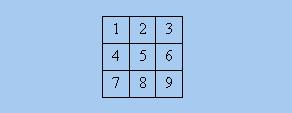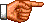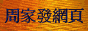# 點算的奧秘：伯恩賽德-波利亞定理

《點算的奧秘：著色問題的基礎知識》中，筆者介紹了求解「著色」問題的基礎知識，其中包括「群」、「陪集」、「群作用」、「穩定集」、「軌道」等重要概念。在本節筆者將介紹如何利用這些概念求解基本的「著色」問題。為方便以下討論，現從上述網頁摘引有關「著色」問題的精確數學定義：

 設有「物件」集合O和「顏色」集合C，並容許O作「運動」(「運動」集合用G表示)，現要用C中元素對O中元素著色，並把「著色」方案表達為從O映射到C的函數(「著色」方案集合用F表示)。如果我們把任何兩個可在某「運動」下互相轉換的「著色」方案視作相同，那麼不同的「著色」方案數目便等於在G「作用」下F的「軌道」數目|F / G|。

f[g*(x)] = g • Stab(x)

|Orb(x)| = |L(x)|    (1)

|G| = |G:Stab(x)| × |Stab(x)|    (2)

|G| = |Orb(x)| × |Stab(x)|    (3) □Orb([o g o g]) = {g**[o g o g]，其中g ∈ D4} = {[o g o g]g*−1，其中g ∈ D4}

[o g o g] r*−1 = [o g o g] [4 1 2 3] = [g o g o]

Orb([o g o g]) = {[o g o g], [g o g o]}

Stab([o g o g]) = {g ∈ D4: g**([o g o g]) = [o g o g]} = {g ∈ D4: [o g o g] g*−1 = [o g o g]}

[o g o g] (r2*)−1 = [o g o g] [3 4 1 2] = [o g o g]

Stab([o g o g]) = {e, r2, m, n}

|F / G| = Σf ∈ F |Stab(f)| / |G|

Σf ∈ Orb(k) |Stab(f)| = |G|    (4)

 Σf ∈ Orb(k) |Stab(f)| = Σf ∈ Orb(k) |G| / |Orb(f)| = Σf ∈ Orb(k) |G| / |Orb(k)| = |Orb(k)| × |G| / |Orb(k)| = |G|

Σf ∈ F |Stab(f)| = |F / G| × |G|

fStab(f)|Stab(f)|
[o o o o]{e, r, r2, r3, v, h, m, n} 8
[o o o g]{e, m}2
[o o g o]{e, n}2
[o o g g]{e, v}2
[o g o o]{e, m}2
[o g o g]{e, r2, m, n}4
[o g g o]{e, h}2
[o g g g]{e, n}2
[g o o o]{e, n}2
[g o o g]{e, h}2
[g o g o]{e, r2, m, n}4
[g o g g]{e, m}2
[g g o o]{e, v}2
[g g o g]{e, n}2
[g g g o]{e, m}2
[g g g g]{e, r, r2, r3, v, h, m, n} 8

Fix(g) = {f ∈ F: g**(f) = f}

|F / G| = Σg ∈ G |Fix(g)| / |G|

Σf ∈ F |Stab(f)| = Σg ∈ G |Fix(g)|    (5)

{(f, g)，其中f ∈ F，g ∈ G，g**(f) = f}

{(g, f)，其中g ∈ G，f ∈ F，g**(f) = f}

D4的元素gg*的「循環式」|Fix(g)|
e(1)(2)(3)(4)24 = 16
r(1 2 3 4)21 = 2
r2(1 3)(2 4)22 = 4
r3(1 4 3 2)21 = 2
v(1 2)(3 4)22 = 4
h(1 4)(2 3)22 = 4
m(1 3)(2)(4)23 = 8
n(1)(2 4)(3)23 = 8

Z[G] = Σg ∈ G Z[g*] / |G|

Z[D4] = (z14 + 2z4 + 3z22 + 2z12z2) / 8

Z[D4](2, 2, 2) = (24 + 2 × 2 + 3 × 22 + 2 × 22 × 2) / 8 = 6

|F / G| = Z[G](c, ... c) □D4的元素gg*的「循環式」Z[g*]
e(1)(2)(3)(4)(5)(6)(7)(8)(9)z19
r(1 7 9 3)(2 4 8 6)(5)z1z42
r2(1 9)(2 8)(3 7)(4 6)(5)z1z24
r3(1 3 9 7)(2 6 8 4)(5)z1z42
v(1 3)(2)(4 6)(5)(7 9)(8)z13z23
h(1 7)(2 8)(3 9)(4)(5)(6)z13z23
m(1)(2 4)(3 7)(5)(6 8)(9)z13z23
n(1 9)(2 6)(3)(4 8)(5)(7)z13z23

Z[D4](z1, z2, z4) = (z19 + 2z1z42 + z1z24 + 4z13z23) / 8

Z[D4](3, 3, 3) = (39 + 2 × 3 × 32 + 3 × 34 + 4 × 33 × 33) / 8 = 2862 □